# Communicating with numbers

## Writing in mathematics

Mathematics writing uses a natural language (i.e. English), symbols, and numbers to communicate with an audience. Problems can occur when you fail to write in sentences and rely heavily on symbols and numbers to communicate with an audience. Using only numbers and symbols in maths communication is the equivalent of writing an essay using only abbreviations, dot points and no punctuation. You need to use words to guide your audience through your explanations.

How to think like a mathematician – Chapters 3 & 4
Kevin Houston

Writing mathematics (these videos are linked to the content in the two chapters above)
Watch part 1
Watch part 2
Check your library or online for a copy of the full book.

## Other maths guides

A guide to writing mathematics
Dr. Kevin Lee

Common errors in writing mathematics
Prof. Stephen B. Maurer

Some remarks on writing mathematical proofs
John M. Lee

Useful mathematical symbols
Wikipedia

Crash course: chemistry
Units and significant figures
Watch video

5 minute physics
Significant figures
View website

# Significant figures

The term ‘significant figures’ is used to describe the digits of numbers that have been measured. The digits communicate important information about the precision of a measurement, regardless of the units. Being able to recognise the number of significant figures is particularly important when you use measurements in calculations or unit conversions.

For example, when using measurements in calculations your final answer will only be as accurate as the LEAST PRECISE measurement. Being able to identify the significant figures in your measurements will ensure you are able to communicate the correct level of precision.

## Significant figures & precision

For every measurement, whether performed by you or a machine, there is estimation involved—you will never be able to measure the EXACT length, weight, or temperature of anything. We can improve the precision of our equipment, but any measurement will only be as precise as the equipment being used to make the measurement (e.g. measuring a distance to the nearest metre, centimetre, or millimetre).

Usually there will be a number (or numbers) that you are certain of plus one digit at the end that is an estimate.

Example

If you write that the length indicated below is 13.2 cm (measured to 3 significant figures), you are saying that you are certain the length is at least 13 cm but you have estimated to the nearest millimetre. The actual length could be anywhere in between 13.1 cm and 13.3 cm.

## General rules for significant figures

The following rules will help you to recognise which numbers are classed as significant figures.

### Digits that are significant

1. All non-zero digits are significant.
Example
8.1 g has 2 significant figures.

2. Zeros between non-zero digits are significant.
Example
60.7 L has 3 significant figures.

3. Trailing zeros to the right of a decimal point are significant.
Example
0.2590 kg has 4 significant figures. This could be written as 259.0 g.

### Digits that aren’t significant

4. Leading zeros to the left of the first non-zero digit are not significant.
Example
0.056 km has 2 significant figures. This could be written as 56 m.

5. Trailing zeros to the left of a decimal point MAY be significant
Example
5600 m could be 2, 3, or 4 significant figures. This depends on whether the measurement was precise to the nearest metre, ten metres, or hundred metres. This uncertainty can be avoided by using scientific notation.
E.g. 5.6 x 103 m (2 significant figures) or 5.600 x 103 m (4 significant figures).

6. Exact numbers have infinite significant figures and they will not limit the accuracy of your calculation.
Exact numbers include counts (e.g. 10 apples) and defined numbers (e.g. 100 centimetres in a metre or the speed of light – 299 792 458 m/s). These numbers are not classed as measurements and significant figures do not need to be considered.

Performing calculations

When you perform a calculation, you must ensure that your final answer presents an appropriate level of precision – don’t just write down all the digits that your calculator displays. The precision of the numbers you put into your calculation will determine the level of precision of your final answer.

When adding or subtracting numbers, your final answer will only be as precise as the LEAST precise value. Instead of counting the number of significant figures, you need to focus on the number of decimal places.

You must ensure that you round off your final answer so it has the same number of decimal places as the value with the fewest decimal places. Make sure that you convert your values to the same units before adding or subtracting.

Addition example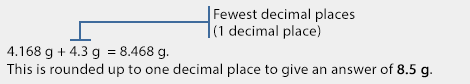Subtraction example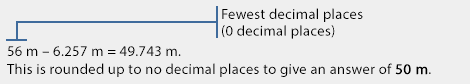## Significant figures for multiplying/dividing

When multiplying or dividing numbers, you need to focus on the number of significant figures rather than the decimal places.

For multiplication and division the fewest significant figures in any number will determine the number of significant figures used in the final answer.

You should refer to the significant rules to ensure you are able to identify the significant figures.

Multiplication example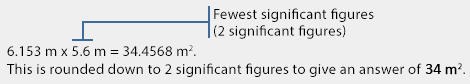Division exampleUnit conversions and SI units

## Unit conversions

Significant figures play an important role in unit conversions. If you’re unable to identify significant figures you run the risk of miscommunicating the level of precision.

Example

Convert 300.0 mm to centimetres.

There are 10 mm in 1 cm, therefore 300.0 mm/10 mm = 30.00 cm.

This answer contains 4 significant figures – the same number found in the original number. It would be incorrect to write 30 cm because you are failing to include the level of precision found in the original number.

*Remember, the 10 mm in this calculation is an exact number and should not be used to determine the significant figures. See rule 6 above.

Example

You take a measurement of the air temperature using a thermometer that measures degrees Fahrenheit. You record a reading of 87 °F. Convert this measurement to degrees Celsius.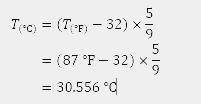This answer is incorrect because it indicates a higher level of precision than what was recorded in the initial measurement.

The correct answer should be rounded off to include 2 significant figures.

Therefore, the correct answer is 31 °C.

## SI units

The International System of Units provides certainty for units of measurement.

### SI prefixes

 Factor Name Symbol Factor Name Symbol 101 deca da 10-1 deci d 102 hector h 10-2 centi c 103 kilo k 10-3 milli m 106 mega M 10-6 micro µ 109 giga G 10-9 nano n 1012 tera T 10-12 pico p 1015 peta P 10-15 femto f 1018 exa E 10-18 atto a 1021 zetta Z 10-21 zepto z 1023 yotta Y 10-23 yocto y
Scientific notation

Scientific notation is a way to display very large and very small numbers in a concise way. From a communication perspective, scientific notation allows you to record measurements in a consistent way for all units and present calculations neatly.

## Scientific notation and significant figures

Using scientific notation can reduce the confusion around identifying the significant figures of a number, particularly when you’re converting to different units.

Example

The length 505.0 m can be expressed as 5.050 x 102 m or:Rounding off

## General rules

1. If the first digit to be dropped is less than 5, drop that digit and all others to the right.
Example
Round 26.7835 to 2 decimal places.
26.7835
26.78

2. If the digit to be dropped is 5 or greater, drop that digit and all others to the right and increase the preceding digit by 1.
Example
Round 4.6887 to 2 decimal places.
4.6887
4.69

Example
Round 35.4253 off to 2 decimal places.
35.4253
35.43

## For large datasets

When rounding off figures in large datasets or spreadsheets, you may need to use the odd-even rule to avoid rounding errors.

### Odd-even rule

If the number to be dropped is 5 and is followed by no digits or only by zeros, apply the odd-even rule.

If the digit before the 5 is even, drop the 5 and any trailing zeros.

Example
Round 12.36500 to 2 decimal places.
12.36500
12.36

If the digit before the five is odd, increase the digit by 1 and drop the 5 and any trailing zeros.

Example
Round 25.73500 to 2 decimal places.
25.73500
25.74

## Multistep calculations

To avoid rounding errors in multistep calculations, you should avoid any rounding off until the final answer. Preferably, you should let your calculator do the work of remembering the details of your calculation and then round off the answer at the end.

If you need to write down answers from your calculator at intermediate steps or record figures in a spreadsheet, you can include “guard digits” to avoid losing information along the way. Guard digits are simply extra digits that you keep at the end of a number to reduce errors caused by rounding off.

For example, at an intermediate step in a calculation your calculator gives you an answer of 56.105763857. If this number should include only 3 decimal places, you could round off the number to include an extra 2 digits to “guard” the number from rounding errors. This would give you the answer of 56.10576 which you could use in the next steps of your calculation.

Example

Calculate the volume of the following rectangular prism using the formula V = L x W x H.

In this example the least precise measurement is 6.1 m (2 significant figures). Therefore, the final answer should have only 2 significant figures.

Full calculation performed on a calculator: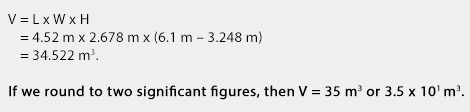Intermediate steps rounded to include up to 2 guard digits:Intermediate steps rounded without guard digits: